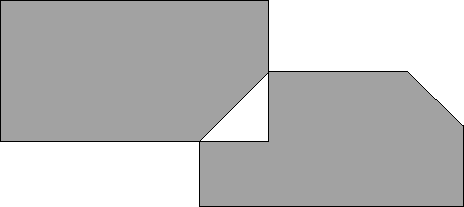# 1088. Polygons

Given two convex polygons, they may or may not overlap. If they do overlap, they will do so to differing degrees and in different ways. Write a program that will read in the coordinates of the corners of two convex polygons and calculate the exclusive or’ of the two areas, that is the area that is bounded by exactly one of the polygons. The desired area is shaded in the following diagram:### 输入格式

Input will consist of pairs of lines each containing the number of vertices of the polygon, followed by that many pairs of integers representing the x,y coordinates of the corners in a clockwise direction. All the coordinates will be positive integers less than 100. For each pair of polygons (pair of lines in the data file), your program should print out the desired area correct to two decimal places. The input will end with a line containing a zero (0).

### 输出格式

Output will consist of a single line containing the desired area written as a succession of eight (8) digit fields with two (2) digits after the decimal point. There will not be enough cases to need more than one line.

### 样例

Input
3 5 5 8 1 2 3
3 5 5 8 1 2 3
4 1 2 1 4 5 4 5 2
6 6 3 8 2 8 1 4 1 4 2 5 3
0

Output
0.00 13.50
`

9 人解决，13 人已尝试。

11 份提交通过，共有 22 份提交。

5.9 EMB 奖励。